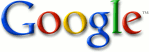SEARCH HOMEMath Central Quandaries & QueriesQuestion from Edward: A Brewer's Sphere that has a 9' Dia.  Holds how much Brew?  How many 40oz bottles will be needed to hold his batch of Brew?  Thanks for the Help.Hi Edward.

The volume of a sphere is calculated with the formula

V = 4/3 π r3.

Find the radius of the sphere and you can use this formula to find the volume in cubic feet.

Then you can convert from cubic feet to ounces using Google (change the 3.3 to what you get):Finally, divide by 40 to find the number of bottles.

Stephen La Rocque. >Math Central is supported by the University of Regina and The Pacific Institute for the Mathematical Sciences.